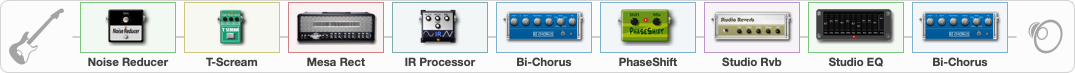# Zakk Wylde - Blood Bath In Paradise

Discussion in 'ToneLib-GFX presets' started by davidtaylors, Jul 30, 2020.

1. ### davidtaylorsWell-Known Member

Zakk Wylde - Blood Bath In Paradise

Preset name: Zakk Wylde - Blood Bath In Paradise

Closest I can get to ZW tone on this song

Effects chain:Effect: "Noise Reducer" (Dynamics / Filter), active - "yes"
{
"Sens" = 88
"Mode" = Soft
}

Effect: "T-Scream" (Overdrive / Distortion), active - "yes"
{
"Drive" = 89
"Tone" = 64
"Level" = 77
}

Effect: "Mesa Rect" (Amp simulators), active - "yes"
{
"Gain" = 47
"Bass" = 73
"Middle" = 76
"Treble" = 70
"Presence" = 81
"Master" = 20
"Level (dB)" = 10
}

Effect: "IR Processor" (Cabinets), active - "yes"
{
"IR" = puppet_stereo_IR
"Low Cut (Hz)" = 0
"Hi Cut (kHz)" = 20.0
"Mix" = 100
"Level (dB)" = 1
}

Effect: "Bi-Chorus" (Modulation / Sfx), active - "yes"
{
"Speed A" = 3.2
"Speed B" = 4.0
"Depth" = 43
"Reso" = 0
"Mode" = Parallel
"Mix" = 56
}

Effect: "PhaseShift" (Modulation / Sfx), active - "yes"
{
"Shift" = 50
"Mix" = 100
}

Effect: "Studio Rvb" (Reverberation), active - "yes"
{
"Time" = 3.4
"PreDelay" = 32
"LoDamp" = 29
"HiDamp" = 94
"Mix" = 73
}

Effect: "Studio EQ" (Dynamics / Filter), active - "yes"
{
"31 Hz" = -8
"62 Hz" = 7
"125 Hz" = 7
"250 Hz" = -5
"500 Hz" = -6
"1 kHz" = -5
"2 kHz" = -7
"4 kHz" = 7
"8 kHz" = 7
"16 kHz" = 0
"Level (dB)" = 6
}

Effect: "Bi-Chorus" (Modulation / Sfx), active - "yes"
{
"Speed A" = 3.2
"Speed B" = 4.0
"Depth" = 43
"Reso" = 0
"Mode" = Parallel
"Mix" = 56
}

Note: You will need to download and install the ToneLib-GFX software to use the preset.